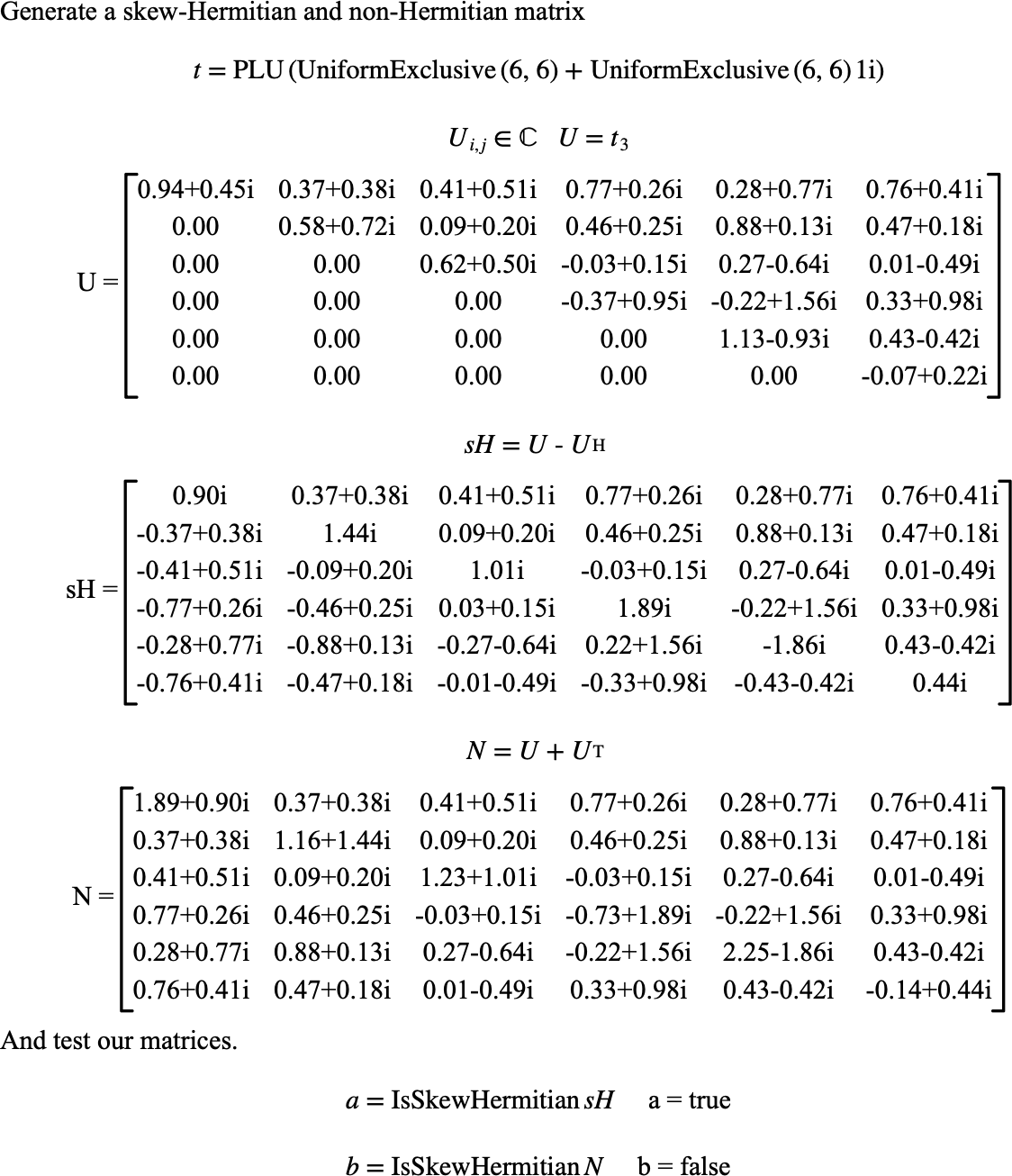# $$\text{IsSkewHermitian}$$¶

You can use the $$\text{IsSkewHermitian}$$ to determine if a matrix is a skew Hermitian matrix.

You can use the \isshermitian backslash command to insert this function.

The following variants of this function are available:

• $$\text{boolean } \text{IsSkewHermitian} \left ( \text{<matrix>} \right )$$

• $$\text{boolean } \text{IsSkewHermitian} \left ( \text{<matrix>}, \text{<relative tolerance>} \right )$$

The $$\text{<matrix>}$$ parameter is the matrix to be tested. The optional $$\text{<relative tolerance>}$$ parameter specifies the tolerance to apply when comparing values. If not specified, the $$\text{IsSkewHermitian}$$ function will use a tolerance of $$1.0 \times 10^{-8}$$.

A matrix, $$M$$, is considered skew Hermitian if $$M _ { i, j } = - \left ( M _ { j, i } \right ) ^ {*}$$. This function relaxes this constraint by allowing a test within a given tolerance. The $$\text{IsSkewHermitian}$$ function considers a matrix to be skew Hermitian if the matrix is square and:

$\left \vert M _ { i,j } + \left ( M _ { j,i } \right ) ^ {*} \right \vert \leq \frac{1}{2} \left \vert M _ { i,j } - \left ( M _ { j,i } \right ) ^ {*} \right \vert t _ {rel}$

For all $$1 \leq i \leq \text{nrows} \left ( M \right )$$ and $$1 \leq j \leq i$$ where $$t _ {rel}$$ is the relative tolerance. Note that this test is only applied if $$M _ { i,j } \ne - \left ( M _ { j,i } \right ) ^ {*}$$.

Figure 160 shows the basic use of the $$\text{IsSkewHermitian}$$ function.Figure 160 Example Use Of The IsSkewHermitian Function